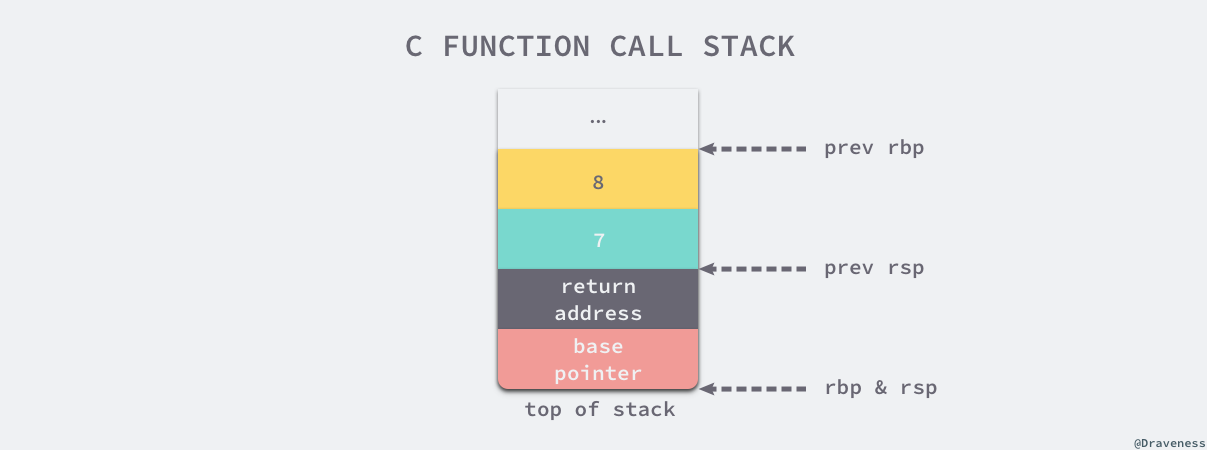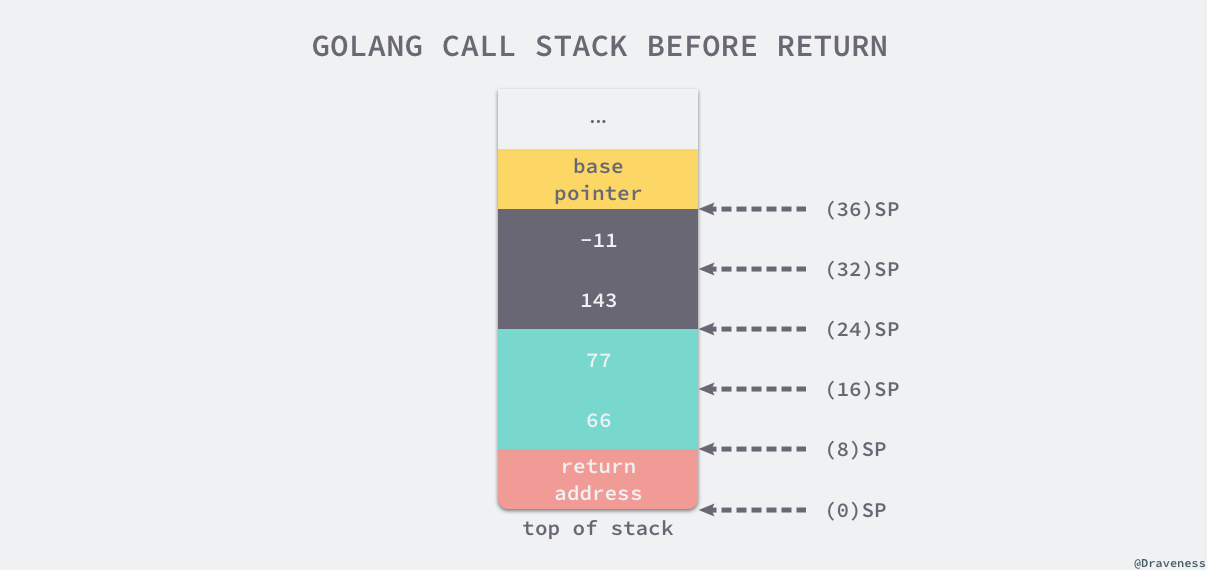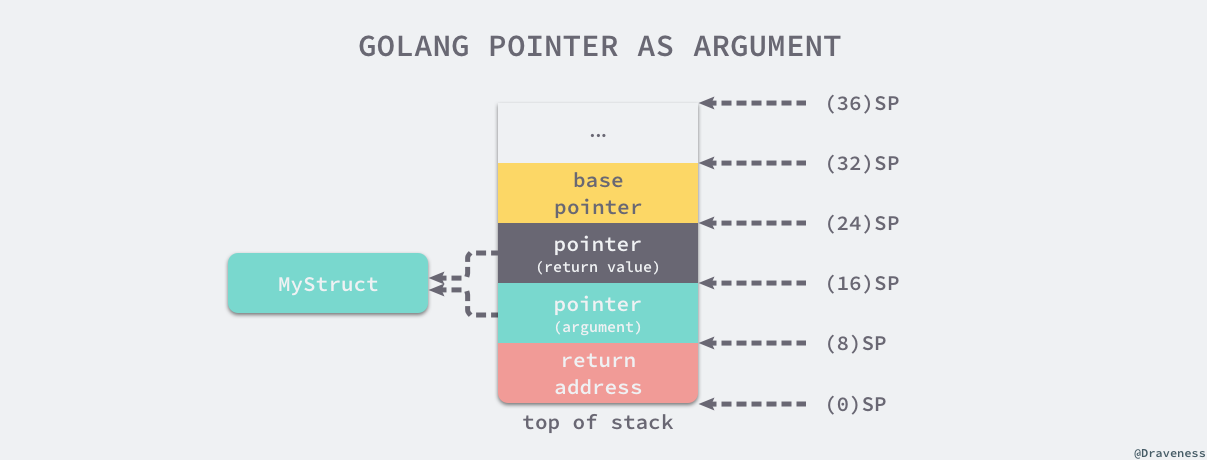# 4.1 函数调用 #

## 4.1.1 调用惯例 #

somefunction(arg0, arg1)


### C 语言 #

gcc 和 clang 编译相同 C 语言代码可能会生成不同的汇编指令，不过生成的代码在结构上不会有太大的区别，所以对只想理解调用惯例的人来说没有太多影响。作者在本节中选择使用 gcc 编译器来编译 C 语言：

$gcc --version gcc (Ubuntu 4.8.2-19ubuntu1) 4.8.2 Copyright (C) 2013 Free Software Foundation, Inc. This is free software; see the source for copying conditions. There is NO warranty; not even for MERCHANTABILITY or FITNESS FOR A PARTICULAR PURPOSE.  假设我们有以下的 C 语言代码，代码中只包含两个函数，其中一个是主函数 main，另一个是我们定义的函数 my_function // ch04/my_function.c int my_function(int arg1, int arg2) { return arg1 + arg2; } int main() { int i = my_function(1, 2); }  我们可以使用 cc -S my_function.c 命令将上述文件编译成如下所示的汇编代码： main: pushq %rbp movq %rsp, %rbp subq$16, %rsp
movl	$2, %esi // 设置第二个参数 movl$1, %edi  // 设置第一个参数
call	my_function
movl	%eax, -4(%rbp)
my_function:
pushq	%rbp
movq	%rsp, %rbp
movl	%edi, -4(%rbp)    // 取出第一个参数，放到栈上
movl	%esi, -8(%rbp)    // 取出第二个参数，放到栈上
movl	-8(%rbp), %eax    // eax = esi = 1
movl	-4(%rbp), %edx    // edx = edi = 2
addl	%edx, %eax        // eax = eax + edx = 1 + 2 = 3
popq	%rbp


• my_function 调用前，调用方 main 函数将 my_function 的两个参数分别存到 edi 和 esi 寄存器中；
• my_function 调用时，它会将寄存器 edi 和 esi 中的数据存储到 eax 和 edx 两个寄存器中，随后通过汇编指令 addl 计算两个入参之和；
• my_function 调用后，使用寄存器 eax 传递返回值，main 函数将 my_function 的返回值存储到栈上的 i 变量中；
int my_function(int arg1, int arg2, int ... arg8) {
return arg1 + arg2 + ... + arg8;
}


main:
pushq	%rbp
movq	%rsp, %rbp
subq	$16, %rsp // 为参数传递申请 16 字节的栈空间 movl$8, 8(%rsp)   // 传递第 8 个参数
movl	$7, (%rsp) // 传递第 7 个参数 movl$6, %r9d
movl	$5, %r8d movl$4, %ecx
movl	$3, %edx movl$2, %esi
movl	$1, %edi call my_function  main 函数调用 my_function 时，前六个参数会使用 edi、esi、edx、ecx、r8d 和 r9d 六个寄存器传递。寄存器的使用顺序也是调用惯例的一部分，函数的第一个参数一定会使用 edi 寄存器，第二个参数使用 esi 寄存器，以此类推。 最后的两个参数与前面的完全不同，调用方 main 函数通过栈传递这两个参数，下图展示了 main 函数在调用 my_function 前的栈信息：图 4-1 C 语言 main 函数的调用栈 上图中 rbp 寄存器会存储函数调用栈的基址指针，即属于 main 函数的栈空间的起始位置，而另一个寄存器 rsp 存储的是 main 函数调用栈结束的位置，这两个寄存器共同表示了函数的栈空间。 在调用 my_function 之前，main 函数通过 subq$16, %rsp 指令分配了 16 个字节的栈地址，随后将第六个以上的参数按照从右到左的顺序存入栈中，即第八个和第七个，余下的六个参数会通过寄存器传递，接下来运行的 call my_function 指令会调用 my_function 函数：

my_function:
pushq	%rbp
movq	%rsp, %rbp
movl	%edi, -4(%rbp)    // rbp-4 = edi = 1
movl	%esi, -8(%rbp)    // rbp-8 = esi = 2
...
movl	-8(%rbp), %eax    // eax = 2
movl	-4(%rbp), %edx    // edx = 1
addl	%eax, %edx        // edx = eax + edx = 3
...
movl	16(%rbp), %eax    // eax = 7
addl	%eax, %edx        // edx = eax + edx = 28
movl	24(%rbp), %eax    // eax = 8
addl	%edx, %eax        // edx = eax + edx = 36
popq	%rbp


my_function 会先将寄存器中的全部数据转移到栈上，然后利用 eax 寄存器计算所有入参的和并返回结果。

• 六个以及六个以下的参数会按照顺序分别使用 edi、esi、edx、ecx、r8d 和 r9d 六个寄存器传递；
• 六个以上的参数会使用栈传递，函数的参数会以从右到左的顺序依次存入栈中；

### Go 语言 #

package main

func myFunction(a, b int) (int, int) {
return a + b, a - b
}

func main() {
myFunction(66, 77)
}


"".main STEXT size=68 args=0x0 locals=0x28
0x0000 00000 (main.go:7)	MOVQ	(TLS), CX
0x0009 00009 (main.go:7)	CMPQ	SP, 16(CX)
0x000d 00013 (main.go:7)	JLS	61
0x000f 00015 (main.go:7)	SUBQ	$40, SP // 分配 40 字节栈空间 0x0013 00019 (main.go:7) MOVQ BP, 32(SP) // 将基址指针存储到栈上 0x0018 00024 (main.go:7) LEAQ 32(SP), BP 0x001d 00029 (main.go:8) MOVQ$66, (SP)    // 第一个参数
0x0025 00037 (main.go:8)	MOVQ	$77, 8(SP) // 第二个参数 0x002e 00046 (main.go:8) CALL "".myFunction(SB) 0x0033 00051 (main.go:9) MOVQ 32(SP), BP 0x0038 00056 (main.go:9) ADDQ$40, SP
0x003c 00060 (main.go:9)	RETmain 函数通过 SUBQ $40, SP 指令一共在栈上分配了 40 字节的内存空间： 空间大小作用 SP+32 ~ BP8 字节main 函数的栈基址指针 SP+16 ~ SP+3216 字节函数 myFunction 的两个返回值 SP ~ SP+1616 字节函数 myFunction 的两个参数 表 4-1 主函数调用栈 myFunction 入参的压栈顺序和 C 语言一样，都是从右到左，即第一个参数 66 在栈顶的 SP ~ SP+8，第二个参数存储在 SP+8 ~ SP+16 的空间中。 当我们准备好函数的入参之后，会调用汇编指令 CALL "".myFunction(SB)，这个指令首先会将 main 的返回地址存入栈中，然后改变当前的栈指针 SP 并执行 myFunction 的汇编指令： "".myFunction STEXT nosplit size=49 args=0x20 locals=0x0 0x0000 00000 (main.go:3) MOVQ$0, "".~r2+24(SP) // 初始化第一个返回值
0x0009 00009 (main.go:3)	MOVQ	$0, "".~r3+32(SP) // 初始化第二个返回值 0x0012 00018 (main.go:4) MOVQ "".a+8(SP), AX // AX = 66 0x0017 00023 (main.go:4) ADDQ "".b+16(SP), AX // AX = AX + 77 = 143 0x001c 00028 (main.go:4) MOVQ AX, "".~r2+24(SP) // (24)SP = AX = 143 0x0021 00033 (main.go:4) MOVQ "".a+8(SP), AX // AX = 66 0x0026 00038 (main.go:4) SUBQ "".b+16(SP), AX // AX = AX - 77 = -11 0x002b 00043 (main.go:4) MOVQ AX, "".~r3+32(SP) // (32)SP = AX = -11 0x0030 00048 (main.go:4) RET  从上述的汇编代码中我们可以看出，当前函数在执行时首先会将 main 函数中预留的两个返回值地址置成 int 类型的默认值 0，然后根据栈的相对位置获取参数并进行加减操作并将值存回栈中，在 myFunction 函数返回之间，栈中的数据如下图所示：图 4-3 myFunction 函数返回前的栈 myFunction 返回后，main 函数会通过以下的指令来恢复栈基址指针并销毁已经失去作用的 40 字节栈内存：  0x0033 00051 (main.go:9) MOVQ 32(SP), BP 0x0038 00056 (main.go:9) ADDQ$40, SP
0x003c 00060 (main.go:9)    RET


### 对比 #

C 语言和 Go 语言在设计函数的调用惯例时选择了不同的实现。C 语言同时使用寄存器和栈传递参数，使用 eax 寄存器传递返回值；而 Go 语言使用栈传递参数和返回值。我们可以对比一下这两种设计的优点和缺点：

• C 语言的方式能够极大地减少函数调用的额外开销，但是也增加了实现的复杂度；
• CPU 访问栈的开销比访问寄存器高几十倍3
• 需要单独处理函数参数过多的情况；
• Go 语言的方式能够降低实现的复杂度并支持多返回值，但是牺牲了函数调用的性能；
• 不需要考虑超过寄存器数量的参数应该如何传递；
• 不需要考虑不同架构上的寄存器差异；
• 函数入参和出参的内存空间需要在栈上进行分配；

Go 语言使用栈作为参数和返回值传递的方法是综合考虑后的设计，选择这种设计意味着编译器会更加简单、更容易维护。

## 4.1.2 参数传递 #

• 传值：函数调用时会对参数进行拷贝，被调用方和调用方两者持有不相关的两份数据；
• 传引用：函数调用时会传递参数的指针，被调用方和调用方两者持有相同的数据，任意一方做出的修改都会影响另一方。

### 整型和数组 #

func myFunction(i int, arr int) {
fmt.Printf("in my_funciton - i=(%d, %p) arr=(%v, %p)\n", i, &i, arr, &arr)
}

func main() {
i := 30
arr := int{66, 77}
fmt.Printf("before calling - i=(%d, %p) arr=(%v, %p)\n", i, &i, arr, &arr)
myFunction(i, arr)
fmt.Printf("after  calling - i=(%d, %p) arr=(%v, %p)\n", i, &i, arr, &arr)
}

$go run main.go before calling - i=(30, 0xc00009a000) arr=([66 77], 0xc00009a010) in my_funciton - i=(30, 0xc00009a008) arr=([66 77], 0xc00009a020) after calling - i=(30, 0xc00009a000) arr=([66 77], 0xc00009a010)  当我们通过命令运行这段代码时会发现，main 函数和被调用者 myFunction 中参数的地址是完全不同的。 不过从 main 函数的角度来看，在调用 myFunction 前后，整数 i 和数组 arr 两个参数的地址都没有变化。那么如果我们在 myFunction 函数内部对参数进行修改是否会影响 main 函数中的变量呢？这里更新 myFunction 函数并重新执行这段代码： func myFunction(i int, arr int) { i = 29 arr = 88 fmt.Printf("in my_funciton - i=(%d, %p) arr=(%v, %p)\n", i, &i, arr, &arr) }$ go run main.go
before calling - i=(30, 0xc000072008) arr=([66 77], 0xc000072010)
in my_funciton - i=(29, 0xc000072028) arr=([66 88], 0xc000072040)
after  calling - i=(30, 0xc000072008) arr=([66 77], 0xc000072010)


### 结构体和指针 #

type MyStruct struct {
i int
}

func myFunction(a MyStruct, b *MyStruct) {
a.i = 31
b.i = 41
fmt.Printf("in my_function - a=(%d, %p) b=(%v, %p)\n", a, &a, b, &b)
}

func main() {
a := MyStruct{i: 30}
b := &MyStruct{i: 40}
fmt.Printf("before calling - a=(%d, %p) b=(%v, %p)\n", a, &a, b, &b)
myFunction(a, b)
fmt.Printf("after calling  - a=(%d, %p) b=(%v, %p)\n", a, &a, b, &b)
}

$go run main.go before calling - a=({30}, 0xc000018178) b=(&{40}, 0xc00000c028) in my_function - a=({31}, 0xc000018198) b=(&{41}, 0xc00000c038) after calling - a=({30}, 0xc000018178) b=(&{41}, 0xc00000c028)  从上述运行的结果我们可以得出如下结论： • 传递结构体时：会拷贝结构体中的全部内容； • 传递结构体指针时：会拷贝结构体指针； 修改结构体指针是改变了指针指向的结构体，b.i 可以被理解成 (*b).i，也就是我们先获取指针 b 背后的结构体，再修改结构体的成员变量。我们简单修改上述代码，分析一下 Go 语言结构体在内存中的布局： type MyStruct struct { i int j int } func myFunction(ms *MyStruct) { ptr := unsafe.Pointer(ms) for i := 0; i < 2; i++ { c := (*int)(unsafe.Pointer((uintptr(ptr) + uintptr(8*i)))) *c += i + 1 fmt.Printf("[%p] %d\n", c, *c) } } func main() { a := &MyStruct{i: 40, j: 50} myFunction(a) fmt.Printf("[%p] %v\n", a, a) }$ go run main.go
[0xc000018180] 41
[0xc000018188] 52
[0xc000018180] &{41 52}


type MyStruct struct {
i int
j int
}

func myFunction(ms *MyStruct) *MyStruct {
return ms
}

$go tool compile -S -N -l main.go "".myFunction STEXT nosplit size=20 args=0x10 locals=0x0 0x0000 00000 (main.go:8) MOVQ$0, "".~r1+16(SP) // 初始化返回值
0x0009 00009 (main.go:9)	MOVQ	"".ms+8(SP), AX   // 复制引用
0x000e 00014 (main.go:9)	MOVQ	AX, "".~r1+16(SP) // 返回引用
0x0013 00019 (main.go:9)	RET## 4.1.3 小结 #

1. 通过堆栈传递参数，入栈的顺序是从右到左，而参数的计算是从左到右；
2. 函数返回值通过堆栈传递并由调用者预先分配内存空间；
3. 调用函数时都是传值，接收方会对入参进行复制再计算；

## 4.1.4 延伸阅读 #

1. GCC, the GNU Compiler Collection https://gcc.gnu.org/ ↩︎

2. Clang: a C language family frontend for LLVM https://clang.llvm.org/ ↩︎

3. Understanding CPU caching and performance https://arstechnica.com/gadgets/2002/07/caching/2/ ↩︎

## 购买纸质书